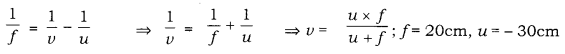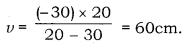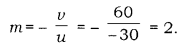# 1. If the image formed by a lens is diminished in size and erect for all positions of the object, what type of lens is it?

1. If the image formed by a lens is diminished in size and erect for all positions of the object, what type of lens is it?
2. Name the point on the lens through which a ray of light passes undeviated.
3. An object is placed perpendicular to the principal axis of a convex lens of focal length 20 cm. The distance of the object from the lens is 30 cm.
Find
(i) the position
(ii) the magnification and
(iii) the nature of the image formed.

1 If the image formed by a lens is diminished in size and erect, for all positions of the object, then the lens is a concave lens.
2.The point on the lens through which a ray of light passes undeviated is known as Pole.3.(i). The image is formed at a distance of 60cm on the other side of the optical centre.
(ii).(iii). Image formed is inverted